# Few Operator used in c++

10. Jan 2018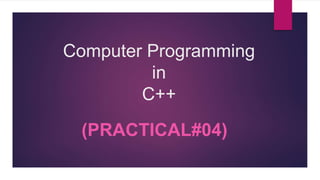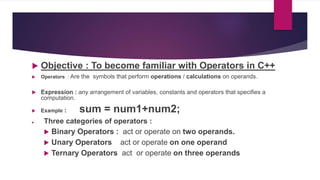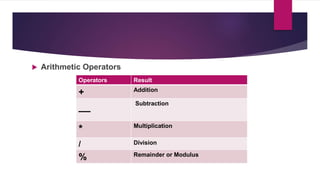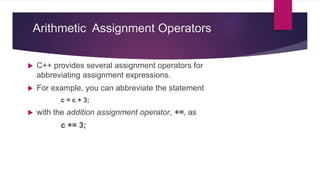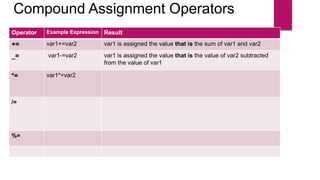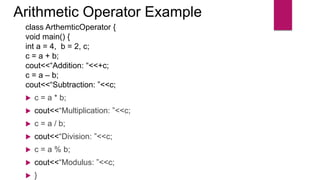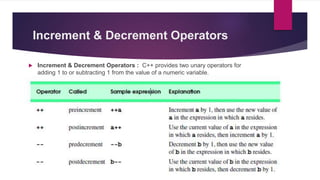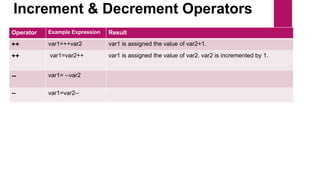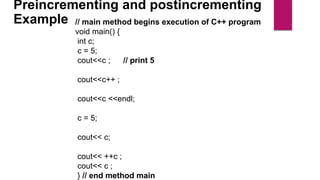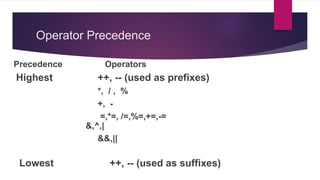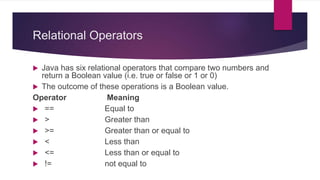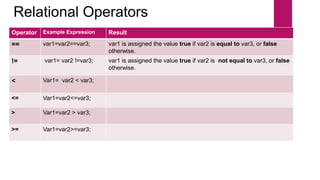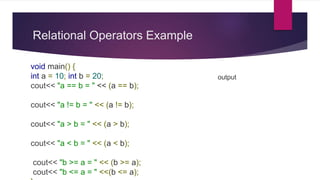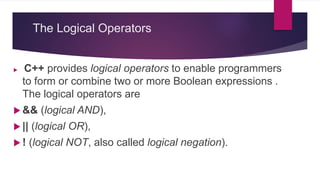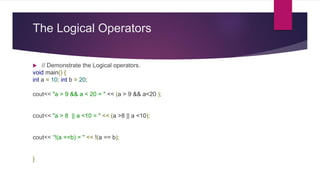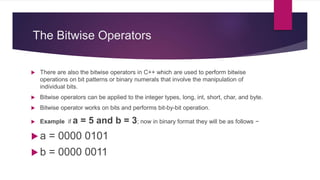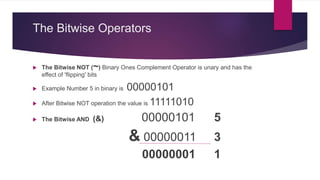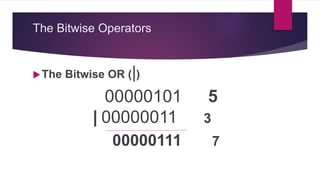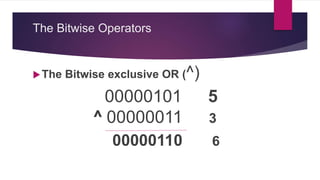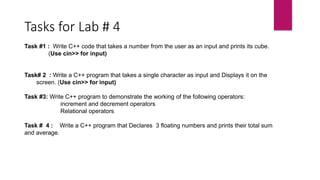1 von 20

### Few Operator used in c++

• 2.  Objective : To become familiar with Operators in C++  Operators : Are the symbols that perform operations / calculations on operands.  Expression : any arrangement of variables, constants and operators that specifies a computation.  Example : sum = num1+num2;  Three categories of operators :  Binary Operators : act or operate on two operands.  Unary Operators act or operate on one operand  Ternary Operators act or operate on three operands
• 3.  Arithmetic Operators Operators Result + Addition __ Subtraction * Multiplication / Division % Remainder or Modulus
• 4. Arithmetic Assignment Operators  C++ provides several assignment operators for abbreviating assignment expressions.  For example, you can abbreviate the statement c = c + 3;  with the addition assignment operator, +=, as c += 3;
• 5. Compound Assignment Operators Operator Example Expression Result += var1+=var2 var1 is assigned the value that is the sum of var1 and var2 _= var1-=var2 var1 is assigned the value that is the value of var2 subtracted from the value of var1 *= var1*=var2 /= %=
• 6. Arithmetic Operator Example class ArthemticOperator { void main() { int a = 4, b = 2, c; c = a + b; cout<<“Addition: “<<+c; c = a – b; cout<<“Subtraction: ”<<c;  c = a * b;  cout<<“Multiplication: ”<<c;  c = a / b;  cout<<“Division: ”<<c;  c = a % b;  cout<<“Modulus: ”<<c;  }
• 7. Increment & Decrement Operators  Increment & Decrement Operators : C++ provides two unary operators for adding 1 to or subtracting 1 from the value of a numeric variable.
• 8. Increment & Decrement Operators Operator Example Expression Result ++ var1=++var2 var1 is assigned the value of var2+1. ++ var1=var2++ var1 is assigned the value of var2. var2 is incremented by 1. -- var1= --var2 -- var1=var2--
• 9. Preincrementing and postincrementing Example // main method begins execution of C++ program void main() { int c; c = 5; cout<<c ; // print 5 cout<<c++ ; cout<<c <<endl; c = 5; cout<< c; cout<< ++c ; cout<< c ; } // end method main
• 10. Operator Precedence Precedence Operators Highest ++, -- (used as prefixes) *, / , % +, - =,*=, /=,%=,+=,-= &,^,| &&,|| Lowest ++, -- (used as suffixes)
• 11. Relational Operators  Java has six relational operators that compare two numbers and return a Boolean value (i.e. true or false or 1 or 0)  The outcome of these operations is a Boolean value. Operator Meaning  == Equal to  > Greater than  >= Greater than or equal to  < Less than  <= Less than or equal to  != not equal to
• 12. Relational Operators Operator Example Expression Result == var1=var2==var3; var1 is assigned the value true if var2 is equal to var3, or false otherwise. != var1= var2 !=var3; var1 is assigned the value true if var2 is not equal to var3, or false otherwise. < Var1= var2 < var3; <= Var1=var2<=var3; > Var1=var2 > var3; >= Var1=var2>=var3;
• 13. Relational Operators Example void main() { int a = 10; int b = 20; cout<< "a == b = " << (a == b); cout<< "a != b = " << (a != b); cout<< "a > b = " << (a > b); cout<< "a < b = " << (a < b); cout<< "b >= a = " << (b >= a); cout<< "b <= a = " <<(b <= a); output
• 14. The Logical Operators  C++ provides logical operators to enable programmers to form or combine two or more Boolean expressions . The logical operators are  && (logical AND),  || (logical OR),  ! (logical NOT, also called logical negation).
• 15. The Logical Operators  // Demonstrate the Logical operators. void main() { int a = 10; int b = 20; cout<< "a > 9 && a < 20 = " << (a > 9 && a<20 ); cout<< "a > 8 || a <10 = " << (a >8 || a <10); cout<< “!(a ==b) = " << !(a == b); }
• 16. The Bitwise Operators  There are also the bitwise operators in C++ which are used to perform bitwise operations on bit patterns or binary numerals that involve the manipulation of individual bits.  Bitwise operators can be applied to the integer types, long, int, short, char, and byte.  Bitwise operator works on bits and performs bit-by-bit operation.  Example if a = 5 and b = 3; now in binary format they will be as follows −  a = 0000 0101  b = 0000 0011
• 17. The Bitwise Operators  The Bitwise NOT (~) Binary Ones Complement Operator is unary and has the effect of 'flipping' bits  Example Number 5 in binary is 00000101  After Bitwise NOT operation the value is 11111010  The Bitwise AND (&) 00000101 5 & 00000011 3 00000001 1
• 18. The Bitwise Operators The Bitwise OR (|) 00000101 5 | 00000011 3 00000111 7
• 19. The Bitwise Operators The Bitwise exclusive OR (^) 00000101 5 ^ 00000011 3 00000110 6
• 20. Tasks for Lab # 4 Task #1 : Write C++ code that takes a number from the user as an input and prints its cube. (Use cin>> for input) Task# 2 : Write a C++ program that takes a single character as input and Displays it on the screen. (Use cin>> for input) Task #3: Write C++ program to demonstrate the working of the following operators: increment and decrement operators Relational operators Task # 4 : Write a C++ program that Declares 3 floating numbers and prints their total sum and average.